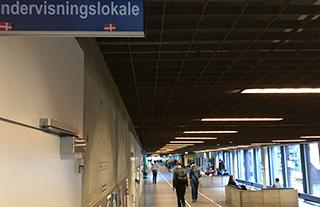# Introductory quantum mechanics II, spring 2017 (FY522: 5 ECTS)The aim of the course is to give the students a basic understanding of the quantum mechanical wave mechanics and its application to different physical phenomena supplemented by an introductory training in the mathematical formalism and problem solving. The course gives an academic basis for further studies in quantum physics, as well as studies among others the topics particle physics and solid state physics, that are placed later in the education. The course is the continuation of FY521. In relation to the competence profile of the degree it is the explicit focus of the course to: (i) give skills to apply physical principles and mathematical tools to formulate and solve physical models; (ii) give knowledge and understanding of quantum mechanics; (iii) give ability to acquire new knowledge in an effective and autonomous way and apply this knowledge reflectively; (iv)give understanding of how scientific knowledge is obtained by an interplay between theory and experiment.

After completing the course the student is expected to be able to:

• apply different analytical methods to characterize simple quantum systems;
• use different abstract formulations of quantum mechanics;
• work with angular momentum;
• perform perturbation calculations;
• use variational methods for approximate calculations;